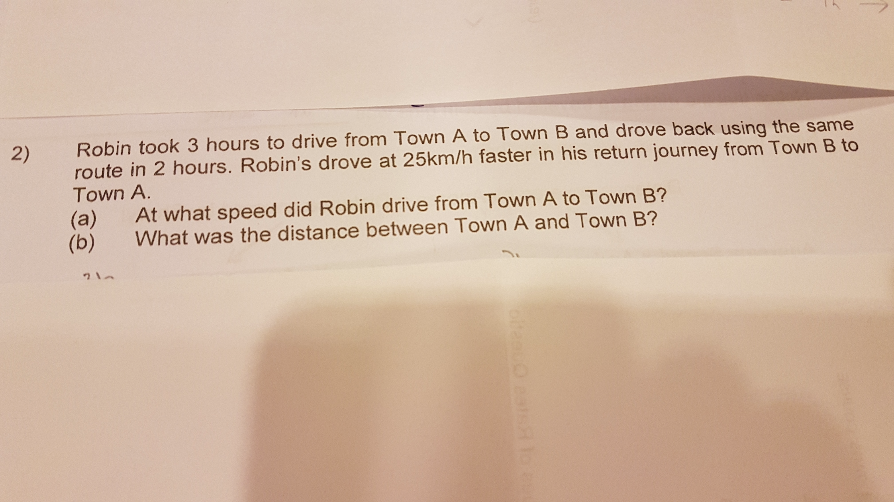# QuestionFrom A to B, 3 hours at speed S for a distance of 3S km.

From B to A, 2 hours at speed (S + 25) for a distance of 2(S + 25) = 2S + 50

Distance is same…

3S = 2S + 50

S = 50 km/h

(a) From A to B, speed -> 50 km/h

(b) Distance -> 50 × 3 = 150 km